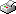Print This Page

Centrality and Random Graphs

Christian Tallberg

Summary

Centrality in random graphs is studied in two reports: Testing Centrality in Random Graphs and Comparing Degree-based and Closeness-based Centrality Measures.

By using block models, centrality is tested in random graphs. The null hypothesis model assumed to generate edges with common probability is the Bernoulli(p) -distribution. First an attempt is made to derive the maximum likelihood estimators of the parameters in the block model. It is shown that they are troublesome to derive. Therefore centrality testing is performed by computer simulation. Properties of the tests are investigated. Actor level centrality indices are aggregated to obtain graph centrality indices. Ten tests based on graph level centralities are presented. Two of the tests are based on degree and eight of the tests are based on closeness. It is concluded that none of the tests are uniformly most powerful. A general tendency is that the heterogeneity- based tests generate stronger power than the average-based tests. Noted is that also the tests defined as the maximums of the actor centralities have strong power.

Under the assumptions that the edges in realizations of directed random graphs are conditionally independent Bernoulli(pi)-distributed, where the edge probabilities, pi, are independently beta-distributed, statistical properties of four graph centrality measures are investigated. Three of the measures are extensively used within the context of social networks; the maximum of the actor centralities, the mean centrality and the variance of the centralities. The fourth measure considered is the difference between the maximum of the actor centralities and the mean centrality. The graph centrality measures are investigated for two different actor centrality concepts, degree centrality and closeness centrality. Analytical results of statistical properties of the measures for degree-based centrality are derived, while similar results for closeness-based measures are difficult to derive. Statistical properties are obtained for both degree-based measures and closeness-based measures by computer simulation, where the empirical results agree with the theoretically derived results for the degree-based measures.